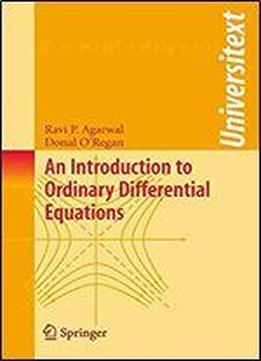# An Introduction To Ordinary Differential Equations (universitext) by Ravi P. Agarwal / 2008 / English / PDF

Ordinary differential equations serve as mathematical models for many exciting real world problems. Rapid growth in the theory and applications of differential equations has resulted in a continued interest in their study by students in many disciplines. This textbook organizes material around theorems and proofs, comprising of 42 class-tested lectures that effectively convey the subject in easily manageable sections. The presentation is driven by detailed examples that illustrate how the subject works. Numerous exercise sets, with an "answers and hints" section, are included. The book further provides a background and history of the subject.

views: 475